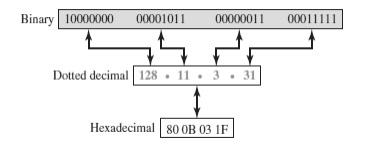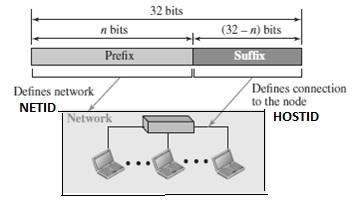The Internet Protocol version 4 (IPv4) is called the fourth version in the development of the Internet Protocol. Its address is a 32-bit address which uniquely defines the connection that means here each address defines one, and only one, connection to the Internet.

If a device has two connections to the Internet, via two networks, then we call it has two IPv4 addresses.

IPv4 addresses are universal in a sense where the addressing system must be accepted by any host that wants to be connected to the Internet.

There are three notations to show an IPv4 address which are as follows −

• binary notation (base 2)
• dotted-decimal notation (base 256)

The three notations to show an IPv4 address are diagrammatically represented below −### Binary notation

Here, IPv4 address is displayed as 32 bits. To make the address more readable, one or more spaces are inserted between bytes (8 bits).

### Dotted-decimal notation

Here, IPv4 addresses are written in decimal form with a decimal point separating the bytes. Each number in the dotted-decimal notation is between 0 and 255.

Each hexadecimal digit is equivalent to four bits. This means a 32-bit address that has 8 hexadecimal digits. This notation is often used in network programming.

The different types of communication network that involve delivery, the addressing system is hierarchical.

A 32-bit IPv4 address is in the form of hierarchical but divided only into two parts as follows −

• The first part of the address is called as the prefix, which defines the network(Net ID).

• The second part of the address is called as the suffix, which defines the node (Host ID).

Usually, the prefix length is n bits and the suffix length is (32-n) bits.

A prefix can be fixed length (or) variable length.The network identifier in the IPv4 was first designed as a fixed-length prefix. This scheme is referred to as classful addressing.

The new scheme, which is referred to as classless addressing, uses a variable length network prefix.